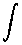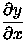# Some mathematical notes

The first part of this page, ``A brief history of calculus'', is a collection of notes I made as a prelude to a History of Science paper that never happened. (I did the Euclid paper instead.)

The second part, ``Other interesting stuff'', is just a collection of miscellaneous events in the history of math.

Citations appear in ``angle-brackets'' (e.g., <TT>). The list of sources appears at the bottom of this page.

## A brief history of calculus

### Ancient notions

c.360 B.C.
Eudoxus of Cnidus provides a ``method of exhaustion'', close to the limiting concept of calculus, which is used by himself and later Greeks to find areas and volumes of curvilinear figures; it was based on the lemma that any non-zero quantity can be made as large as one wishes by multiplying it by a large enough constant. <HM>

### Pre-calculus

1615
Johannes Kepler uses infinitesimals to calculate volumes of revolution in Nova stereometria doliorum vinarorum (``New Measurement of the Volume of Wine Casks''). <TT>
1635
Bonaventura Cavalieri calculates volumes using infinitely small sections. <TT>
1655
John Wallis studies infinite series in Arithmetica infinitorum (``Arithmetic of Infinitesimals'') <TT>
1658
Blaise Pascal, working on the sine function, ``almost'' discovers calculus. <TT>

### Early calculus

1665
Isaac Newton retires to the country to escape the Great Plague in London; there he invents the first form of calculus. <TT>
1668
James Gregory includes a geometrical version of the fundamental theorem of calculus in Geometriae pars universalis, inserviens quantitatum curvarum transmutationi & measurae (``Geometrical Exercises and the Universal Part of Geometry''). <TT>
1669
Newton includes his method for finding areas under curves in his De analysi per equationes numero terminorum infinitas (``On the Analysis of Equations Unlimited in the Number of Their Terms''), circulated privately. <TT>
1670
Isaac Barrow uses methods similar to calculus to draw tangents to curves, find the lengths of curves, and the areas bounded by curves. <TT>
1675
Gottfried Leibniz introduces the modern notationfor integration and the notation dx/dy for differentiation; he also determines the product rule for differentiation. <TT/MN>
1676
Newton writes two letters to Leibniz, hinting at his work with infinite series and fluxions (his form of calculus); also this year, Leibniz discovers how to differentiate any fractional power of x. <TT>
1677
Leibniz finds the quotient rule for differentiation. <TT>

### Big-time calculus

1684
Leibniz publishes ``A new method for maxima and minima as well as tangents, which is impeded neither by fractional nor by irrational quantities, and a remarkable type of calculus for this''; although only six pages long, few can understand it. <TT>
1686
Leibniz publishes his method of integral calculus in an issue of Acta Eruditorum (``Learned Works''? -- my own translation, so might not be completely satisfactory). <TT>
1691
Michel Rolle states without proof the theorem named after him. <TT>
1693
John Wallis publishes Newton's method of fluxions in volume two of his Opera Mathematica (``Mathematical Works''). <TT>
1694
Jean Bernoulli discovers the method known as l'Hôpital's Rule (a.k.a., l'Hospital's Rule); it is known by that name because Marquis Antoine de l'Hôpital bought it from Bernoulli and introduced it in his influential 1696 textbook Analyse des infiniment petits pour l'Intelligence des Lignes Courbes (``Analysis of Infinitesimals for Understanding Curved Lines''?? -- my own translation). <TT>
1715
Brook Taylor introduces his famous series in Methodus incrementorum directa et inversa (``Direct and Inverse Incremental Method''), in which he develops the calculus of finite differences. <TT>

### Later calculus

1797
Joseph-Louis Lagrange introduces the notations f'x and y' for the derivatives of f(x) and y, respectively. <MN/TT>
1800
Louis F. A. Arbogast introduces the symbol D for the operation of differentiation. <MN/DC>
1841
Carl Gustav Jacob Jacobi adopts the modern notationfor partial differentiation; Adrien-Marie Legendre originally introduced it in 1786, but immediately abandoned it. <MN/TT>
1854
Bernhard Riemann defines the integral in a way that does not require continuity. <TT>
1872
H. Eduard Heine, a student of Karl Weierstrass, presents the modern ``epsilon-delta'' definition of a limit in his Die Elemente der Functionenlehre (``Elements of the Study of Functions''?? -- my own translation). <TT>

## Other interesting stuff

### 2000 B.C. to 200 B.C.

c.1975 B.C.
Mesopotamian mathematicians discover how to solve quadratic equations. <TT>
c.1850 B.C.
Mesopotamian mathematicians discover the so-called Pythagorean theorem approximately 12 centuries before the time of Pythagoras. <TT>
c.465 B.C.
The Pythagorean Hippasus of Metapontum discovers the dodecahedron, a regular solid whose 12 faces are regular pentagons. There are only 4 other regular solids: the tetrahedron (4 equilateral triangles), the cube (6 squares), the octahedron (8 equilateral triangles), and the icosahedron (20 equilateral triangles). <TT/CR/HM>c.450 B.C.
c.350 B.C.
Menaechmus discovers the conic sections: the parabola, ellipse, and hyperbola. <HM>
c.300 B.C.
The thirteen books of Euclid's Elements (the most widely read textbook ever written) contains propositions on plane geometry, the distributive, commutative, and associative laws of arithmetic, quadratic equations, prime numbers (including the theorem that the set of primes is infinite), perfect numbers, greatest common divisors, geometric series, irrational numbers, and solid geometry, including the five regular solids. Unlike the modern treatment of these ideas, the Greeks used an entirely geometrical approach in mathematics, using line segments to represent all magnitudes (numbers). Although the Elements compiles already-known Greek mathematics, some of the proofs are probably Euclid's own. <HM>
c.250 B.C.
Archimedes of Syracuse and the quadrature of the parabola. <HM>

### 1500 A.D. to present

1575
The first known proof by mathematical induction is included in Francesco Maurolico's Arithmeticorum Libri Duo; he proves that the sum of the first n odd integers is n^2. <TT>
1635
Rene Descartes discovers that any simple convex polyhedron having V vertices, E edges, and F faces obeys the rule V - E + F = 2; since his discovery is not published until 1860, the theorem is named after Leonhard Euler, who rediscovered it in 1752. <TT>
1679
Gottfried Leibniz introduces binary arithmetic in a letter written to Joachim Bouvet, showing that any number may be expressed by 0's and 1's only. <TT>
1781
Joseph-Louis Lagrange expresses in a letter to his mentor Jean le Rond d'Alembert his fear that no further progress can be made in mathematics; despite this dire prophesy, many of his own contributions are still to come. <TT>
1843
While strolling along the Royal Canal, Sir William Rowan Hamilton devises a mathematical system which is not commutative; he develops his idea into a system of ``quaternions'', similar to that of three-dimensional vectors. His ideas help to usher in modern abstract algebra. <TT/HM>
1844
Hermann Gunther-Grassman publishes The Study of Extensions, which deals with multidimensional vectors; he almost single-handedly creates modern linear algebra. <TT/HM>
1865
August Ferdinand Mobius unveils his single-sided, single-edged figure, the Mobius strip. <TT>
1877
Georg Cantor proves that the number of points on a line segment is the same as the number of points in the interior of a square, publication of the result is delayed for a year because mathematicians refuse to believe it. <TT>
1890
Giuseppe Peano discovers a one-dimensional, continuous curve that passes through all the points in the interior of a square. <TT>
1902
Bertrand Russell discovers his ``great paradox''. <TT>
1908
The final edition of Giuseppe Peano's Mathematical Formulas contains about 4,200 theorems. <TT>
1976
Haken, Appel, and Koch prove with the use of a computer that only four colors are required to color in any two-dimensional map in such a way that no two adjacent regions share the same color; this was conjectured in 1850 by Francis Guthrie. <TT>

## Sources

<CR>
CRC Standard Mathematical Tables, 26th edition - William H. Beyer, ed. (CRC Press, 1981)
<DC>
The Historical Development of the Calculus - C. H. Edwards, Jr. (Springer-Verlag, 1979)
<MN>
A History of Mathematical Notations/Volume II: Notations Mainly in Higher Mathematics - Florian Cajori (Open Court Publishing, 1952)
<HM>
A History of Mathematics, second edition - Carl B. Boyer and Uta C. Merzbach (John Wiley & Sons, 1989)
<HC>
The History of the Calculus and Its Conceptual Development - Carl D. Boyer (Dover Publications, 1959)
<TT>
The Timetables of Science: A Chronology of the Most Important People and Events in the History of Science - Alexander Hellemans and Bryan Bunch (Simon & Schuster, 1991)